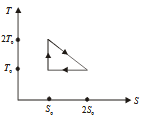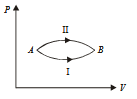## Filters

Sort by :
Clear All
Q
Engineering
381 Views   |

Which of the following is incorrect regarding the first law of thermodynamics?

• Option 1)

It introduces the concept of the internal energy

• Option 2)

It introduces the concept of entropy

• Option 3)

It is not applicable to any cyclic process

• Option 4)

It is a restatement of the principle of conservation of energy

As we learnt in  First law of Thermodynamics - Heat imported to a body in is in general used to increase internal energy and work done against external pressure. - wherein   and   Second Law of Thermodynamics - Planck's Statement - wherein It is impossible to extract heat from single body and convert whole into work.   and   Entropy - It is a measure of disorder of molecular motion of a...
Engineering
1027 Views   |

The temperature­- entropy diagram of a reversible engine cycle is given in the figure. Its efficiency is• Option 1)

• Option 2)

• Option 3)

• Option 4)

As we learnt in  Entropy - It is a measure of disorder of molecular motion of a system. - wherein Greater is disorder greater is entropy and  Efficiency of a cyclic process -   - wherein Gross heat implied only part of heat absorbed.   The efficiency of cycle is: Efficiency        Option 1) This option is correct. Option 2) This option is incorrect. Option 3) This option is...
Engineering
148 Views   |

A system goes from A to B via two processes I and II as shown in figure. If    and are the changes  in internal energies in the processes I and II respectively, then• Option 1)

• Option 2)

• Option 3)

• Option 4)

relation between   can not be determined

As we learnt in  Property of internal energy - U (internal energy) is a state function. - wherein i.e. It depends only on initial and final state.   Change in internal energy depends only at initial and final stage.      Option 1) This option is incorrect. Option 2) This option is incorrect. Option 3) This option is correct. Option 4) relation between   can not be determined This option is...
Engineering
121 Views   |

A gaseous mixture consists of 16 g of helium and 16 g of oxygen. The ratio of the mixture is

• Option 1)

• Option 2)

• Option 3)

• Option 4)

As we learnt in  Specific heat in isobaric process -   - wherein is degree of freedom          Option 1) This option is incorrect. Option 2) This option is incorrect. Option 3) This option is incorrect. Option 4) This option is correct.
Exams
Articles
Questions